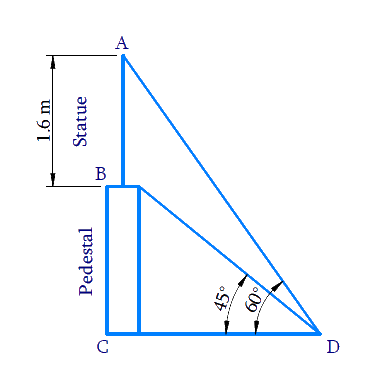# Ex.9.1 Q8 Some Applications of Trigonometry Solution - NCERT Maths Class 10

Go back to  'Ex.9.1'

## Question

A statue, $$1.6\,\rm{m}$$ tall, stands on the top of a pedestal, from a point on the ground. The angle of elevation of the top of the statue is $$60^\circ$$ and from the same point the angle of elevation of the top of the pedestal is $$45^\circ$$. Find the height of the pedestal.

Video Solution
Some Applications Of Trigonometry
Ex 9.1 | Question 8

## Text Solution

What is Known?

(i) Height of statue $$= 1.6 \,\rm{m}$$

(ii) Angle of elevation from ground to top of the statue $$= 60^\circ$$

(iii) Statue stands on the top of the pedestal.

(iv) Angle of elevation of the top of the pedestal (bottom of the statue) $$= 45^\circ$$

What is Unknown?

Height of the pedestalReasoning:

Let the height of the pedestal is $$BC$$, height of the statue, stands on the top of the pedestal, is $$AB$$. $$D$$ is the point on the ground from where the angles of elevation of the bottom $$B$$ and the top $$A$$ of the statue $$AB$$ are $$45^\circ$$ and $$60^\circ$$respectively.

The distance of the point of observation $$D$$ from the base of the pedestal $$C$$ is $$CD$$

Combined height of the pedestal and statue $$AC = AB + BC$$

Trigonometric ratio involving sides $$AC,\, BC, \,CD$$ and $$∠D$$ ($$45^\circ$$and $$60^\circ$$) is $$\tan \theta$$

Steps:

In $$\Delta BCD$$,

\begin{align}\tan {45^0} &= \frac{{BC}}{{CD}}\\1 &= \frac{{BC}}{{CD}}\\BC &= CD\quad\dots \left( {\rm{i}} \right)\end{align}

In  $$\Delta {ACD}$$

\begin{align}\tan {\rm{ }}{60^0} &= \frac{{AC}}{{CD}}\\\tan {\rm{ }}{60^0} &= \frac{{AB + BC}}{{CD}}\\\sqrt 3 &\!=\!\!\frac{{1.6 + BC}}{{BC}}\left[ {{\rm{from}}\left( {\rm{i}} \right)} \right]\\\sqrt 3 BC &= 1.6 + BC\\\sqrt 3 BC - BC &= 1.6\\BC\left( {\sqrt 3 - 1} \right) &= 1.6\\BC = \frac{{1.6}}{{\sqrt 3 - 1}} &\times \frac{{\sqrt 3 + 1}}{{\sqrt 3 + 1}}\\&= \frac{{1.6\left( {\sqrt 3 + 1} \right)}}{{3 - 1}}\\&= \frac{{1.6\left( {\sqrt 3 + 1} \right)}}{2}\\&= 0.8\left( {\sqrt 3 + 1} \right)\end{align}

Height of pedestal $$BC$$ $$=0.8(\sqrt{3}+1) \mathrm{m}$$

Learn from the best math teachers and top your exams

• Live one on one classroom and doubt clearing
• Practice worksheets in and after class for conceptual clarity
• Personalized curriculum to keep up with school